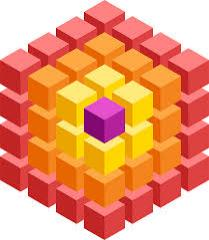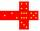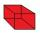# The cube

The cube has a surface area of 486 m ^ 2. Calculate its volume.

V =  729 m3

### Step-by-step explanation:Did you find an error or inaccuracy? Feel free to write us. Thank you!Tips to related online calculators
Tip: Our volume units converter will help you with the conversion of volume units.

## Related math problems and questions:

• Area to volumeIf the surface area of a cube is 486, find its volume.
• The cubeThe cube has a surface of 600 cm2. What is its volume?
• Cube V2SThe volume of the cube is 27 dm cubic. Calculate the surface of the cube.
• The cubeThe cube has a surface area of 216 dm2. Calculate: a) the content of one wall, b) edge length, c) cube volume.
• Cube surfce2volumeCalculate the volume of the cube if its surface is 150 cm2.
• TerezaThe cube has an area of base 256 mm2. Calculate the edge length, volume, and area of its surface.
• The cubeThe surface of the cube is 150 square centimeters. Calculate: a- the content of its walls b - the length of its edges
• Special cubeCalculate the edge of cube, if its surface and its volume is numerically equal number.
• Cuboid to cubeA cuboid with dimensions of 9 cm, 6 cm, and 4 cm has the same volume as a cube. Calculate the surface of this cube.
• Cube 5The content area of one cube wall is 32 square centimeters. Determine the length of its edges, its surface and volume.
• Cube basicsHow long is the edge length of a cube with volume 15 m3?
• Edges or sidesCalculate the cube volume, if the sum of the lengths of all sides is 276 cm.
• Volume and areaWhat is the volume of a cube which has an area of 361 cm2?
• The schoolyardThe schoolyard had the shape of a square with an 11m side. The yard has been enlarged by 75 m2 and has a square shape again. How many meters was each side of the yard enlarged?
• Cube surface and volumeFind the surface of the cube with a volume of 27 dm3.
• Cube 6Volume of the cube is 216 cm3, calculate its surface area.
• Rectangular cuboidThe rectangular cuboid has a surface area 5334 cm2, and its dimensions are in the ratio 2:4:5. Find the volume of this rectangular cuboid.